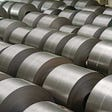# Tutorial on Keras flow_from_dataframe

`pip uninstall keras-preprocessing`
`pip install git+https://github.com/keras-team/keras-preprocessing.git`

# Let’s dive into the code!

`from keras.models import Sequential#Import from keras_preprocessing not from keras.preprocessingfrom keras_preprocessing.image import ImageDataGeneratorfrom keras.layers import Dense, Activation, Flatten, Dropout, BatchNormalizationfrom keras.layers import Conv2D, MaxPooling2Dfrom keras import regularizers, optimizersimport pandas as pdimport numpy as npdef append_ext(fn):    return fn+".png"traindf=pd.read_csv(“./trainLabels.csv”,dtype=str)testdf=pd.read_csv("./sampleSubmission.csv",dtype=str)traindf["id"]=traindf["id"].apply(append_ext)testdf["id"]=testdf["id"].apply(append_ext)datagen=ImageDataGenerator(rescale=1./255.,validation_split=0.25)`
`train_generator=datagen.flow_from_dataframe(dataframe=traindf,directory="./train/",x_col="id",y_col="label",subset="training",batch_size=32,seed=42,shuffle=True,class_mode="categorical",target_size=(32,32))valid_generator=datagen.flow_from_dataframe(dataframe=traindf,directory="./train/",x_col="id",y_col="label",subset="validation",batch_size=32,seed=42,shuffle=True,class_mode="categorical",target_size=(32,32))test_datagen=ImageDataGenerator(rescale=1./255.)test_generator=test_datagen.flow_from_dataframe(dataframe=testdf,directory="./test/",x_col="id",y_col=None,batch_size=32,seed=42,shuffle=False,class_mode=None,target_size=(32,32))`
`model = Sequential()model.add(Conv2D(32, (3, 3), padding='same',                 input_shape=(32,32,3)))model.add(Activation('relu'))model.add(Conv2D(32, (3, 3)))model.add(Activation('relu'))model.add(MaxPooling2D(pool_size=(2, 2)))model.add(Dropout(0.25))model.add(Conv2D(64, (3, 3), padding='same'))model.add(Activation('relu'))model.add(Conv2D(64, (3, 3)))model.add(Activation('relu'))model.add(MaxPooling2D(pool_size=(2, 2)))model.add(Dropout(0.25))model.add(Flatten())model.add(Dense(512))model.add(Activation('relu'))model.add(Dropout(0.5))model.add(Dense(10, activation='softmax'))model.compile(optimizers.rmsprop(lr=0.0001, decay=1e-6),loss="categorical_crossentropy",metrics=["accuracy"])`
`STEP_SIZE_TRAIN=train_generator.n//train_generator.batch_sizeSTEP_SIZE_VALID=valid_generator.n//valid_generator.batch_sizeSTEP_SIZE_TEST=test_generator.n//test_generator.batch_sizemodel.fit_generator(generator=train_generator,                    steps_per_epoch=STEP_SIZE_TRAIN,                    validation_data=valid_generator,                    validation_steps=STEP_SIZE_VALID,                    epochs=10)`

## Evaluate the model

`model.evaluate_generator(generator=valid_generator,steps=STEP_SIZE_TEST)`

## Predict the output

`test_generator.reset()pred=model.predict_generator(test_generator,steps=STEP_SIZE_TEST,verbose=1)`
`predicted_class_indices=np.argmax(pred,axis=1)`
`labels = (train_generator.class_indices)labels = dict((v,k) for k,v in labels.items())predictions = [labels[k] for k in predicted_class_indices]`
`filenames=test_generator.filenamesresults=pd.DataFrame({"Filename":filenames,                      "Predictions":predictions})results.to_csv("results.csv",index=False)`

--

--

--

Machine Learning Enthusiast

Love podcasts or audiobooks? Learn on the go with our new app.

## Application of time series analysis and machine learning to predict length of stay in pediatric…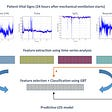## Would this clothing fit me?## One Class Learning in Manufacturing: Autoencoder and Golden Units Baselining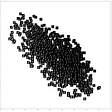## Predict House Price in King County with Azure Machine Learning and Power BI (Part 2)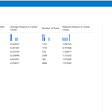## On-Policy v. Off-Policy Reinforcement Learning Explained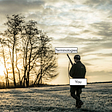## Learn NLP the Stanford way — Lesson 1## Gentle Intro to Machine Learning for Product Managers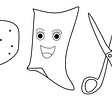## NLP Pipeline 101 With Basic Code Example — Feature ExtractionMachine Learning Enthusiast

## Studying Cross Transferability of Vision Transformers using HAM10000 skin cancer dataset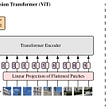## Neural Networks: the best open-source library for object detection & classification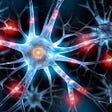## Image Detection with AI Explainability Project## Metric Learning for Steel Surface Classification — Part 1